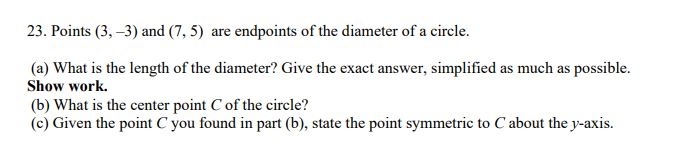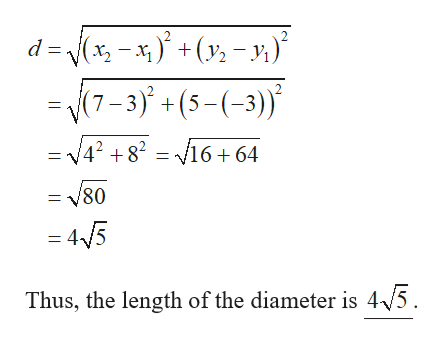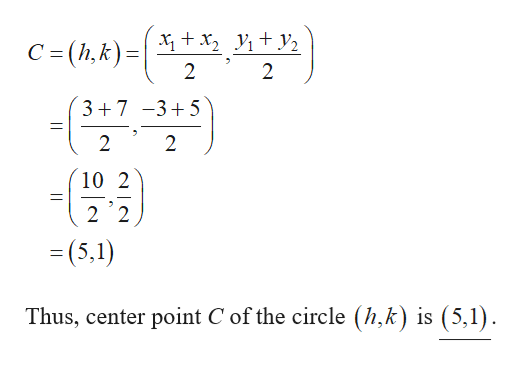# 23. Points (3, –3) and (7, 5) are endpoints of the diameter of a circle.(a) What is the length of the diameter? Give the exact answer, simplified as much as possible.Show work.(b) What is the center point C of the circle?(c) Given the point C you found in part (b), state the point symmetric to Cabout the y-axis.

Question
16 views

practice quuestion 23help_outlineImage Transcriptionclose23. Points (3, –3) and (7, 5) are endpoints of the diameter of a circle. (a) What is the length of the diameter? Give the exact answer, simplified as much as possible. Show work. (b) What is the center point C of the circle? (c) Given the point C you found in part (b), state the point symmetric to Cabout the y-axis. fullscreen
check_circle

Step 1

The given endpoints are (3, –3) and (7,5).

Let (3, –3) be (x1, y1) and (7,5) be (x2, y2).

(a) The length of the diameter is,help_outlineImage Transcriptionclosed = (x, - x, )' +(y; -,)* -/(7-3)° + (5-(-3)) = V4? + 8? = /16 + 64 /80 = 4/5 Thus, the length of the diameter is 4/5. fullscreen
Step 2

(b) The center point C of ...help_outlineImage TranscriptioncloseX + x, V, + y2 C = (h,k)=| (3+7 -3+5 10 2 = (5,1) Thus, center point C of the circle (h,k) is (5,1). fullscreen

### Want to see the full answer?

See Solution

#### Want to see this answer and more?

Solutions are written by subject experts who are available 24/7. Questions are typically answered within 1 hour.*

See Solution
*Response times may vary by subject and question.
Tagged in

### Other